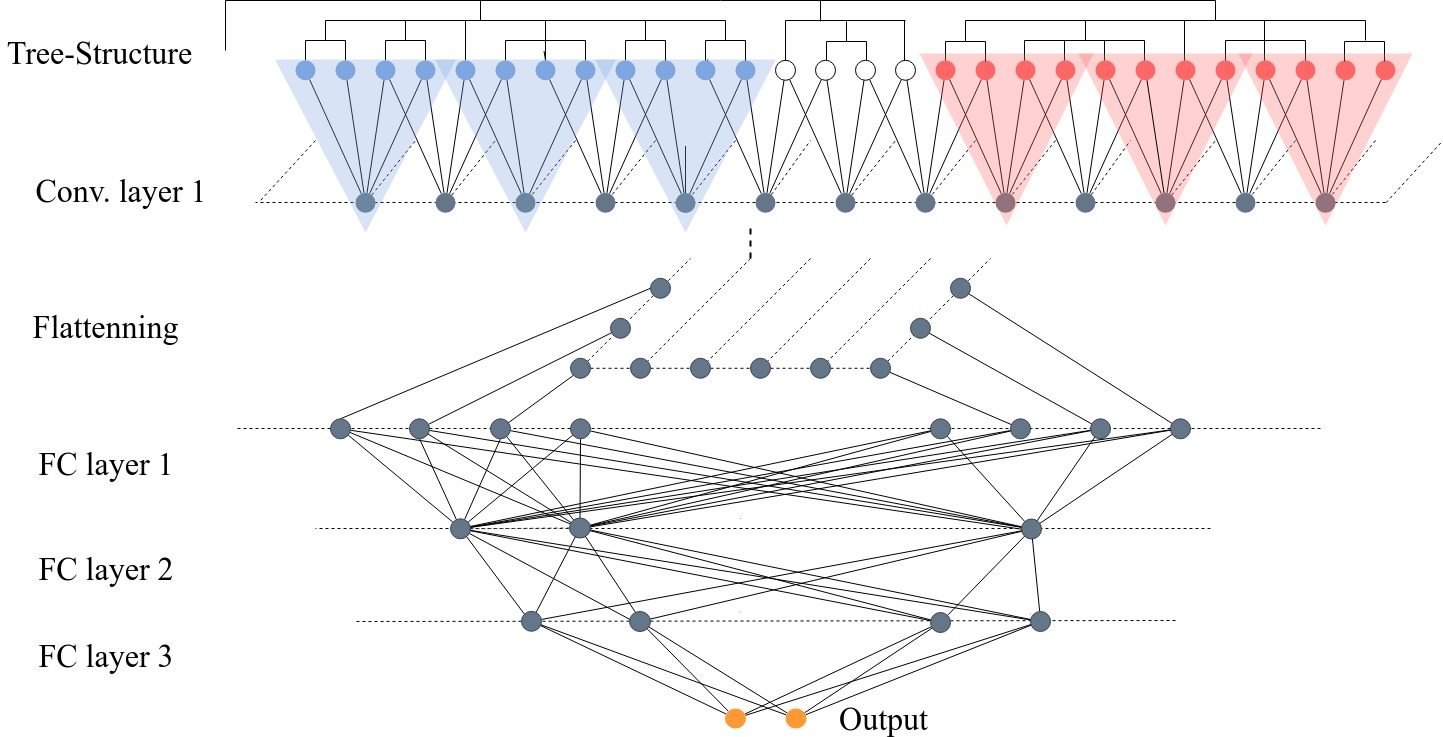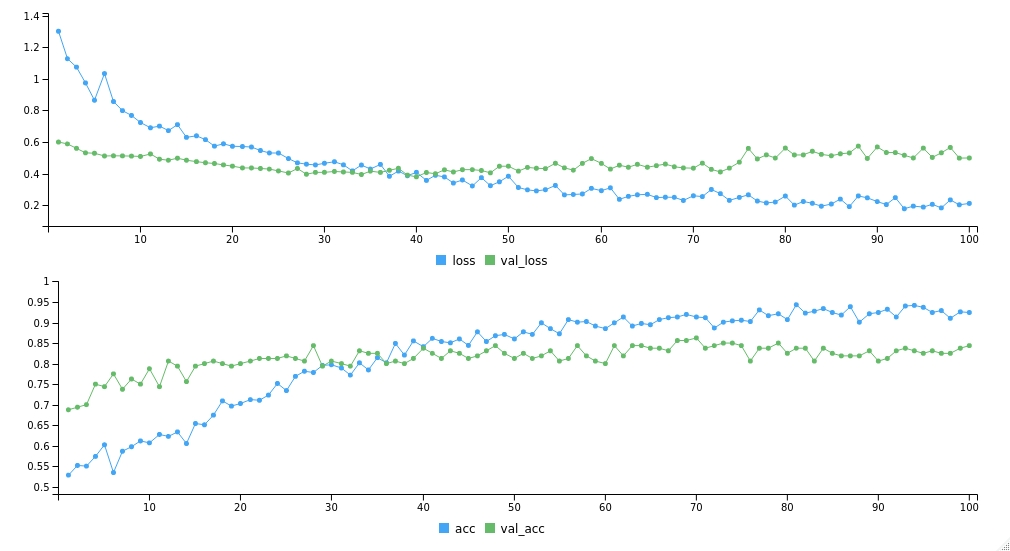# README.md In alfredyewang/tCNN: Tree-regularized Convolutional NeuralNetwork

## Tree-regularized Convolutional NeuralNetwork

We develop a deep learning prediction method "Tree-regularized convolutional Neural Network,"(tCNN) for microbiome-based prediction. The advantage of tCNN is that it uses the convolutional kernel to capture the signals of microbiome species with close evolutionary relationship in a local receptive field. To incorporate the phylogenetic information via the convolutional operation, we adopt the hierarchical agglomerative clustering (HAC) algorithm to cluster the OTUs based on their phylogenetic correlation, making phylogenetically related OTUs close to each other and irrelevant OTUs far apart from each other. For details of pCNN, users can refer to our paper "A Phylogeny-regularized Convolutional NeuralNetwork for Microbiome-based Predictionsn".## Requirements

tCNN is implemented by Keras for R. Please check the guide on official website for detail instruction of installing Keras for R. To use tCNN, R version >= 3.0 is required. Versions for other packages are suggested.

• R >= 3.0 (64-bit)
• Python 2.7 (64-bit)
• Keras 2.2.4
• TensorFlow 1.14.0
• cluster 2.1.0
• dendextend 1.12.0

## Installation

``````devtools::install_github("rstudio/keras")
devtools::install_github("alfredyewang/tCNN")
library("tCNN")
``````

## Usage

``````tCNN(x_train,y_train,x_test,y_test,
C,nCluster,num_classes,
batch_size,epochs,num_filters,window_size,strides_size,
dropout_rate,fc1_units,fc1_activate_function,
fc2_units,fc2_activate_function,
fc3_units,fc3_activate_function
)

``````

## Arguments

| Arguments | Description | | ------------- | ------------- | | x_train |The training dataset| | y_train |The label of training dataset| | x_test | The testing dataset| | y_test |The label of testing dataset| |num_classes |The number of classes| |C | The correlation matrix for Tree Structure | |batch_size | The batch size for neural network | |epochs | The max epoch batch size for training neural network | |num_filters | The number of filters in convolutional layers | |window_size | The window size in convolutional layers| |strides_size | The strides size in convolutional layers | |dropout_rate | The dropout rate for training neural network |fc1_units | The number of node in the first fully connected layers | |fc1_activate_function | The activation function for the first fully connected layers (relu, tanh, softmax)| |fc2_units | The number of node in the second fully connected layers | |fc2_activate_function |The activation function for the second fully connected layers (relu, tanh, softmax)|

## Example

We use use the gut microbiome data collected from twin pairs in Malawi affected by kwashiorkor as an example. These gut microbiome is also profiled using 16S rRNA gene-targeted sequencing and deposited in Qiita with study ID 737. The dataset is downloaded and processed, resulting in a a total of 1041 twins (including MZ and DZ) consisting of 483 females, 512 males and 46 samples with missing gender information profiled with 4321 OTUs. After the same data pre-processing steps aforementioned, we have 995 individuals profiled with 2291 OTUs for the classification task. The detailed pre-processing steps can be found in our paper.

``````library(tCNN)
library(cluster)
library(dendextend)
library(keras)
# Get raw data from twinsgut dataset
``````

Splitting data 8-2 training set and testing set

``````cut = as.integer(dim(z)*0.8)
x_train = z[1:cut,]
y_train = y[1:cut,]
x_test = z[(cut+1):dim(z),]
y_test = y[(cut+1):dim(z),]
``````

Call tCNN package

``````# Call tCNN package
tCNN(x_train=x_train,y_train=y_train,x_test=x_test,y_test=y_test,C=C,num_classes=2,batch_size=16,epochs=100,num_filters=64,window_size=256,strides_size=32,conv1_activate_function='relu',dropout_rate=0.8,fc1_units=128,fc1_activate_function='tanh',fc2_units=32,fc2_activate_function='tanh')
``````

The checkpoint files and model are saved on root directory of tCNN project.#### The tCNN is supported by Google Summer of Code 2019 (R project for statistical computing). Thanks for Google!

alfredyewang/tCNN documentation built on Nov. 2, 2019, 1:40 p.m.